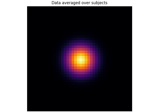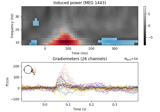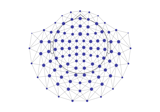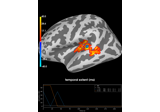Create a sparse binary adjacency/neighbors matrix.

Parameters:
*structure`list`

The adjacency along each dimension. Each entry can be:

• ndarray or scipy.sparse.spmatrix

A square binary adjacency matrix for the given dimension. For example created by `mne.channels.find_ch_adjacency()`.

• int

The number of elements along the given dimension. A lattice adjacency will be generated, which is a binary matrix reflecting that element N of an array is adjacent to elements at indices N - 1 and N + 1.

Returns:
adjacency`scipy.sparse.coo_matrix`, shape (n_features, n_features)

The square adjacency matrix, where the shape `n_features` corresponds to the product of the length of all dimensions. For example `len(times) * len(freqs) * len(chans)`.

Notes

For 4-dimensional data with shape `(n_obs, n_times, n_freqs, n_chans)`, you can specify no connections among elements in a particular dimension by passing a matrix of zeros. For example:

```>>> import numpy as np
>>> from scipy.sparse import diags
>>> n_times, n_freqs, n_chans = (50, 7, 16)
>>> chan_adj = diags([1., 1.], offsets=(-1, 1), shape=(n_chans, n_chans))
...     n_times,  # regular lattice adjacency for times
...     np.zeros((n_freqs, n_freqs)),  # no adjacency between freq. bins
...     )
<5600x5600 sparse matrix of type '<class 'numpy.float64'>'
with 27076 stored elements in COOrdinate format>
```

## Examples using `mne.stats.combine_adjacency`#Statistical inference

Statistical inferenceNon-parametric 1 sample cluster statistic on single trial power

Non-parametric 1 sample cluster statistic on single trial powerSpatiotemporal permutation F-test on full sensor data

Spatiotemporal permutation F-test on full sensor dataPermutation t-test on source data with spatio-temporal clustering

Permutation t-test on source data with spatio-temporal clustering